# Will I ever be a bayesian statistician ? (part 1)

Last week, during the workshop on Statistical Methods for Meteorology and Climate Change (here), I discovered how powerful bayesian techniques could be, and that there were more and more bayesian statisticians. So, if I was to fully understand applied statisticians in conferences and workshops, I really have to understand basics of bayesian statistics. I have published some time ago some posts on bayesian statistics applied to actuarial problems (here or there), but so far, I always thought that bayesian was a synonym for magician. To be honest, I am a Muggle, and I have not been trained as a bayesian. But I can be an opportunist…

So I decided to publish some posts on bayesian techniques, in order to prove that it is actually not that difficult to implement.As far as I understand it, in bayesian statistics, the parameter is considered as a random variable (which is also the case, in classical mathematical statistics). But here, here assume that this parameter does have a parametric distribution….
Consider a classical statistical problem: assume we have a sample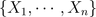i.i.d. with distribution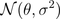. Here we note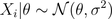since parameteris a random variable. The idea is to assume thathas a (so called a priori) distribution, e.g.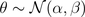So far it was simple. The idea is then to consider the posterior distribution of, given the observations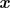. Thus, we need to compute the distribution of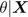which is here extremely simple (due to properties of the Gaussian distribution), i.e.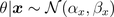where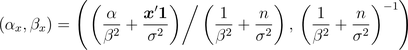And them, it becomes extremely natural to consider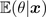as an estimator of given our sample data (and thus, we also have a confidence interval since we know the distribution ofgiven the observations).
In order to be sure that we understood, consider now a heads and tails problem, i.e.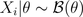. Note, first, that \theta has support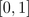. So we need a distribution on that support. Why not a beta distribution ? E.g.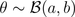Thus,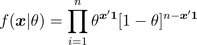and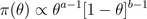From Bayes formula,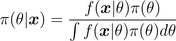and we get easily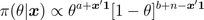which is the density of a Beta distribution, i.e.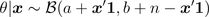prior=dbeta(u,a,b)
posterior=dbeta(u,a+y,n-y+b)

The estimator proposed is then the expected value of that conditional distribution,Note that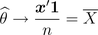Further, it is possible to derive confidence intervals using quantiles of the posterior distribution.
On the graphs below, we consider the following heads/tails sampleA first idea is to consider a uniform prior distribution.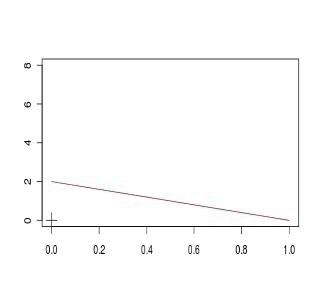A second idea is to consider an asymmetric beta distribution. First, with an asymmetry on the left,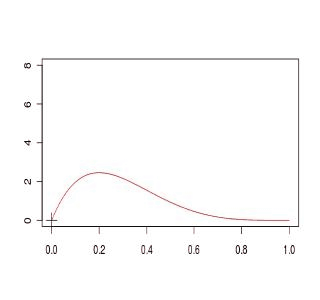or on the right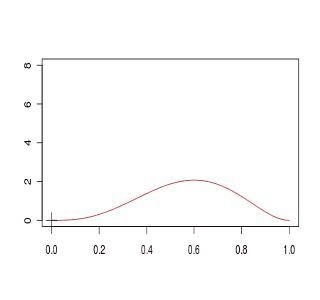Finally a third idea is simply to get back to the standard Gaussian approximation,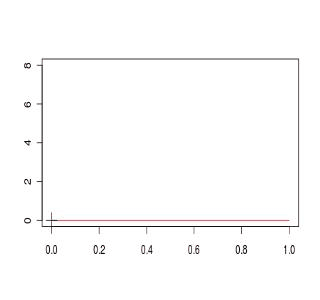If we compare the four models, we obtain (the plain black line is the Gaussian approximated distribution for the empirical mean), and red lines are obtained from prior beta distributionsThe code to generate those graphs is the following
a1=1; b1=1
D1[1,]=dbeta(u,a,b)
a2=4; b2=2
D2[1,]=dbeta(u,a,b)
a3=2; b3=4
D3[1,]=dbeta(u,a,b)
setseed(1)
S=sample(0:1,size=100,replace=TRUE)
COULEUR=rev(rainbow(120))
D1=D2=D3=D4=matrix(NA,101,length(u))
for(s in 1:100){
y=sum(S[1:s])
D1[s+1,]=dbeta(u,a1+y,s-y+b1)
D2[s+1,]=dbeta(u,a2+y,s-y+b2)
D3[s+1,]=dbeta(u,a3+y,s-y+b3)
D4[s+1,]=dnorm(u,y/s,sqrt(y/s*(1-y/s)/s))
plot(u,D1[1,],col="black",type="l",ylim=c(0,8),
xlab="",ylab="")
for(i in 1:s){lines(u,D1[1+i,],col=COULEUR[i])}
points(y/s,0,pch=3,cex=2)
plot(u,D2[1,],col="black",type="l",ylim=c(0,8),
xlab="",ylab="")
for(i in 1:s){lines(u,D2[1+i,],col=COULEUR[i])}
points(y/s,0,pch=3,cex=2)
plot(u,D3[1,],col="black",type="l",ylim=c(0,8),
xlab="",ylab="")
for(i in 1:s){lines(u,D3[1+i,],col=COULEUR[i])}
points(y/s,0,pch=3,cex=2)
plot(u,D4[1,],col="white",type="l",ylim=c(0,8),
xlab="",ylab="")
for(i in 1:s){lines(u,D4[1+i,],col=COULEUR[i])}
points(y/s,0,pch=3,cex=2)
plot(u,D4[s+1,],col="black",lwd=2,type="l",
ylim=c(0,8),xlab="",ylab="")
lines(u,D1[1+i,],col="blue")
lines(u,D2[1+i,],col="red")
lines(u,D3[1+i,],col="purple")
points(y/s,0,pch=3,cex=2)
}

Here, we can see that computations are simple if the prior distribution has a distribution which is the conjugate of the observations’ distribution (see here for the list of prior and posterior standard distributions).
So far, I have two questions that naturally show up

• is it possible to start with a neutral prior distribution, non informative ?
• what if we are no longer working with conjugate distributions ?

Well, I guess I have to work a bit more to answer those questions…. to be continued## 5 thoughts on “Will I ever be a bayesian statistician ? (part 1)”

1.Maria says:

Bonjour, j’apprends à utiliser R et j’ai essayé de lancer votre code pour le graphique mais malheureusement je n’arrive pas de lui faire marcher. Pourriez-vous préciser s’il y a besoin de packages ou de library spécieux pour que ça marche? Merci

2.Washington S, Silva says:

I think the correct is that, in Bayesian inference, the uncertainty about the parameter is represented by a random variable, not the parameter itself. Congratulations on the excellent blog.

1.Arthur Charpentier says:

thanks… and as mentioned in the title, additional posts are in progress…

3.Manoel Galdino says:

Nice post.

1. As far as I know, there is a looong discussion about non-informative prior and objective prios. Take a look on Jefrey’s prios for mor abou objective (or non informative) priors.

The problem é that there isn’t any neutral prior. Sometimes it may be an imporper prior, but this can cause problems…

2. Non-conjugate… well, that’s easy and we just need to say MCMC. It’s possible to ample from the posterior distributions if we can sampreo from conditionals. Even if we are not able to integrate it, we still cam sample usin Monte carlo Simulation.

Nowadays Gibbs Sampler and Metropolis-Hasting algorithm are classics of Bayesian computation.

Last, but not least, one of the best features of Bayesian statistics is hierarchical models.

Take a look at Andrew Gelman’s blog and you will find a lot of interesting stuff about this.

best regards,

Manoel Galdino

4.Thomas says:

La roue tourne horizontalement bien sûr !

😉

This site uses Akismet to reduce spam. Learn how your comment data is processed.